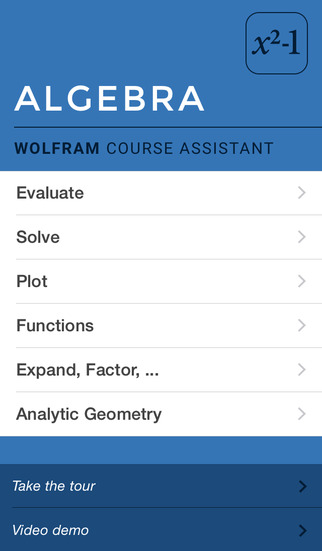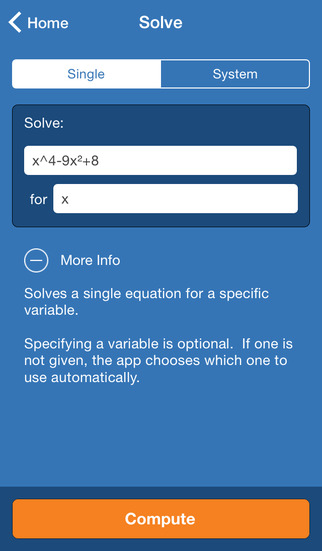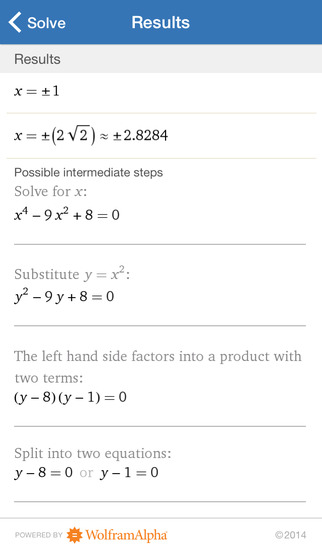5.0

# Wolfram Algebra Course Assistant

• 内容摘要
Taking algebra? Then you need the Wolfram Algebra Course Assistant. This definitive app for algebra—from the world leader in math software—will help you quickly solve your homework problems, ace your tests, and learn algebra concepts so you're prepared for your next courses. Forget canned examples! The Wolfram Algebra Course Assistant solves your specific algebra problems on the fly, often showing you how to work through the problem step by step.

This app covers the following topics applicable to Algebra I, Algebra II, and College Algebra:

- Evaluate any numeric expression or substitute a value for a variable.
- Simplify fractions, square roots, or any other expression.
- Solve a simple equation or a system of equations for specific variables.
- Plot basic, parametric, or polar plots of the function(s) of your choice.
- Expand any polynomial.
- Factor numeric expressions, polynomials, and symbolic expressions.
- Divide any two expressions.
- Find the partial fraction decomposition of rational expressions.

The Wolfram Algebra Course Assistant is powered by the Wolfram|Alpha computational knowledge engine and is created by Wolfram Research, makers of Mathematica—the world's leading software system for mathematical research and education.

The Wolfram Algebra Course Assistant draws on the computational power of Wolfram|Alpha's supercomputers over a 3G, 4G, or Wi-Fi connection.

展开↓

• 版本中的新功能

展开↓

• 应用截图••#### 同类热门推荐

••Toca Life: Hospital 1.0.1 | 220.00MB
••iTunes U 3.6 | 56.30MB
•Toca Life: Office 1.0.1 | 228.00MB

#### 开发商的其他应用

•WolframAlpha 1.7.3 | 30.40MB
••••#### 不可错过# 安装同步助手后能更便捷的下载此应用• 免费下载游戏和应用
• 快速安装APP
• 便捷管理、优化手机
A# 连接设备至同步助手，自动安装“Wolfram Algebra Course Assistant”

### 安装步骤• 色情内容
• 赌博
• 暴力内容
• 反动内容
• 侵犯版权
• 木马病毒
• 恶意扣费
• 不良插件
• 无法安装或者启动
• 其他